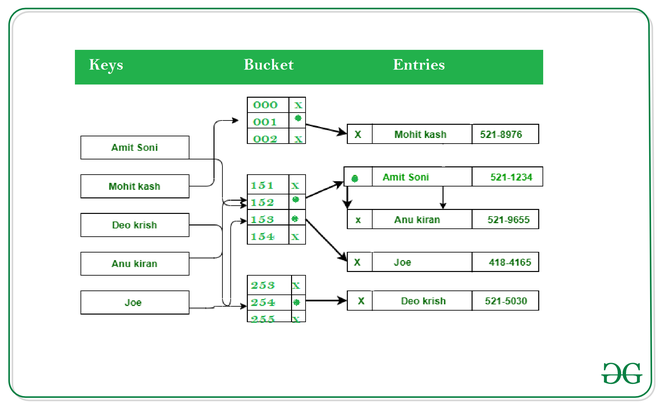# Internal Structure of Python Dictionary

• Last Updated : 22 Nov, 2021

Dictionary in Python is an unordered collection of data values, used to store data values like a map, which unlike other Data Types that hold only a single value as an element, Dictionary holds key:value pair. Key-value is provided in the dictionary to make it more optimized

The dictionary consists of a number of buckets. Each of these buckets contains the hash code of the object that contains the key-value pair. A pointer to the key object and a pointer to the value object.

The below diagram shows the internal structure of the dictionary:The dictionary starts with 8 empty buckets. This is then resized by doubling the number of entries whenever its capacity is reached. This sums up to at least 12 bytes on a 32bit machine and 24 bytes on a 64bit machine

Example 1: An empty python dictionary consumes 240 bytes

## Python3

 `# code` `import` `sys`   `d ``=` `{}` `print``(sys.getsizeof(d))`

Output:

`240`

Example 2. The first time we create a dictionary it contains only 8 slots that can be filled with key-value pairs.

## Python3

 `# code` `import` `sys`   `d ``=` `{}` `d[``'python'``] ``=` `1` `print``(sys.getsizeof(d))`

Output:

`240`

As you can see the size of the dictionary is still the same after adding something to it. The dictionary stores in the bucket which is not full yet.

Example 3:

The key-values not stored in the dictionary itself the size of the dictionary doesn’t change even if we increase the size of its value

## Python3

 `import` `sys`   `d ``=` `{}` `d[``'a'``] ``=` `'a'` `*` `100000` `print``(``"Size of dictionary ->"``, sys.getsizeof(d))` `print``(``"Size of a ->"``, sys.getsizeof(``'a'``))`

Output

```Size of dictionary -> 240
Size of a -> 50```

Output:

```Size of dictionary -> 240
Size of a -> 50```

Example 4: If we remove the items from a dictionary the size of the dictionary will still be the same.

## Python3

 `# code` `import` `sys`   `d ``=` `{}` `d[``'python'``] ``=` `1` `for` `key ``in` `list``(d.keys()):` `  ``d.pop(key)` `  `  `print``(``len``(d))` `print``(sys.getsizeof(d))`

Output:

```0
240```

Here, you can see the dictionary hasn’t released the memory it has allocated. It removes the reference from the hash table but the value is in the memory. Since it is not allocated may become part of garbage collection.

Example 5: If we empty the dictionary using the clear method the size of it is 72 bytes less than the initialized empty dictionary i.e. 240 bytes

## Python3

 `import` `sys`   `d ``=` `{}` `d[``'python'``] ``=` `1` `for` `key ``in` `list``(d.keys()):` `  ``d.pop(key)` `  `  `print``(``len``(d))` `d.clear()` `print``(sys.getsizeof(d))`

Output:

```0
72```

This is because the method clears up memory. It also clears the initial default space i.e., 8 buckets allocated within the dictionary.

My Personal Notes arrow_drop_up
Recommended Articles
Page :